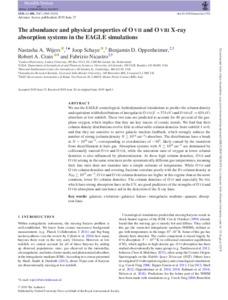# The abundance and physical properties of O VII and O VIII X-ray absorption systems in the EAGLE simulations

Wijers, NA, Schaye, J, Oppenheimer, BD, Crain, RA and Nicastro, F (2019) The abundance and physical properties of O VII and O VIII X-ray absorption systems in the EAGLE simulations. Monthly Notices of the Royal Astronomical Society, 488 (2). p. 2947. ISSN 0035-8711Preview
Text
stz1762.pdf - Published Version

We use the EAGLE cosmological, hydrodynamical simulations to predict the column density and equivalent width distributions of intergalactic O VII ($E=574$ eV) and O VIII ($E=654$ eV) absorbers at low redshift. These two ions are predicted to account for 40% of the gas-phase oxygen, which implies that they are key tracers of cosmic metals. We find that their column density distributions evolve little at observable column densities from redshift 1 to 0, and that they are sensitive to AGN feedback, which strongly reduces the number of strong (column density $N \gtrsim 10^{16} \, \mathrm{cm}^{-2})$ absorbers. The distributions have a break at $N \sim 10^{16} \, \mathrm{cm}^{-2}$, corresponding to overdensities of $\sim 10^{2}$, likely caused by the transition from sheet/filament to halo gas. Absorption systems with $N \gtrsim 10^{16} \mathrm{cm}^{-2}$ are dominated by collisionally ionized O VII and O VIII, while the ionization state of oxygen at lower column densities is also influenced by photoionization. At these high column densities, O VII and O VIII arising in the same structures probe systematically different gas temperatures, meaning their line ratio does not translate into a simple estimate of temperature. While O VII and O VIII column densities and covering fractions correlate poorly with the H I column density at $N_{\mathrm{H \, I}} \gtrsim 10^{15} \, \mathrm{cm}^{-2}$, O VII and O VIII column densities are higher in this regime than at the more common, lower H I column densities. The column densities of O VI and especially Ne VIII, which have strong absorption lines in the UV, are good predictors of the strengths of O VII and O VIII absorption and can hence aid in the detection of the X-ray lines.View Item